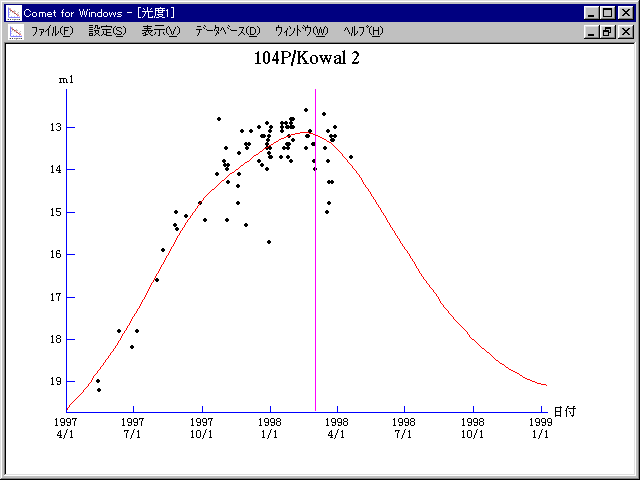# \$B%3%o%kBh#2WB@1(B

104P/Kowal 2 (1998)###\$B50F;MWAG(B

```   The following improved orbital elements by Kenji Muraoka,
are from 422 observations 1991 to 1998, including 9 Planets,
Moon and 5 minor planets perturbations and non-gravitational
effect of style II.  The mean residual is +/- 0.89 arc seconds.

Epoch  =  1998 Mar.  8.0  TT       JDT = 2450880.5
T  =  1998 Mar.  2.15401       +/- 0.00141 (m.e.) TT
Peri. =  191.89338                +/- 0.00107
Node  =  246.14703                +/- 0.00008   (2000.0)
Incl. =   15.48916                +/- 0.00001
q  =    1.3966251              +/- 0.0000040 AU
e  =    0.5852289              +/- 0.0000082
a  =    3.3672193              +/- 0.0000200 AU
n  =    0.15951333             +/- 0.00000142
P  =    6.179                  +/- 0.0000551  years
A1  =   +4.220                  +/- 0.256
A2  =   -0.10338                +/- 0.09889
```

###\$B@1?^(B1997\$BG/(B 3\$B7n(B 3\$BF|!A(B1998\$BG/(B 4\$B7n(B27\$BF|(B1998\$BG/(B 4\$B7n(B27\$BF|!A(B1999\$BG/(B 6\$B7n(B21\$BF|(B

###\$B8wEYJQ2=(B

```        m1 = 10.3 + 5 log\$B&\$(B + 12.5 log r
```##### \$B50F;MWAG\$OB<2,7r<#;a\$N7W;;\$K\$h\$k\$b\$N\$G\$9!#(B \$B@1?^\$O(B StellaNavigator Ver.2.0 for Windows (\$B%"%9%H%m%"!<%D(B \$BJTCx(B / \$B%"%9%-!<=PHG6I4)(B) \$B\$G:n@.\$7\$?\$b\$N\$G\$9!#(B \$B8wEY%0%i%U\$O(BComet for Windows\$B\$G:n@.\$7\$?\$b\$N\$G\$9!#(B